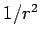Next: Gauss' Law Up: Gauss' Law Previous: Gauss' Law

## Electric Field-Lines

An electric field can be represented diagrammatically as a set of lines with arrows on, called electric field-lines, which fill space. Electric field-lines are drawn according to the following rules:
The direction of the electric field is everywhere tangent to the field-lines, in the sense of the arrows on the lines. The magnitude of the field is proportional to the number of field-lines per unit area passing through a small surface normal to the lines.
Thus, field-lines determine the magnitude, as well as the direction, of the electric field. In particular, the field is strong at points where the field-lines are closely spaced, and weak at points where they are far apart.The electric field-lines associated with a positive point charge are a set of unbroken, evenly spaced (in solid angle) straight-lines which radiate from the charge--see Fig. 9. Thus, the tangent to the field-lines is always directed radially away from the charge, giving the correct direction for the electric field. The number of electric field-lines per unit area normal to the lines falls off like, whereis the radial distance from the charge, since the total number of lines is fixed, whereas the area normal to the lines increases like. Thus, the electric field-strength falls off like, in accordance with Coulomb's law.

By analogy, the electric field-lines associated with a negative point charge are a set of unbroken, evenly spaced (in solid angle) straight lines which converge on the charge.

As a general rule, electric field-lines generated by fixed charges begin on positive charges, end on negative charges, and are unbroken and never cross in the vacuum regions between charges.Next: Gauss' Law Up: Gauss' Law Previous: Gauss' Law
Richard Fitzpatrick 2007-07-14